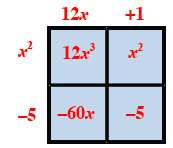### Home > CCAA8 > Chapter 3 Unit 4 > Lesson CCA: 3.2.4 > Problem3-70

3-70.

Use a generic rectangle to multiply the following expressions. Write each solution both as a sum and as a product. Homework Help ✎

1. $(2x+5)(x+6)$

• Draw a generic rectangle and label the length and width.

$(2x+5)(x+6)=2x^2+17x+30$

 $2x$ $+5$ $x$ $+6$

 $2x$ $+5$ $x$ $2x^2$ $5x$ $+6$ $12x$ $30$

Fill in the grid by multiplying the length and width of each box.

Write an equation showing the product is the sum of the parts.
Area as a sum: $2x^2+12x+5x+30=2x^2+17x+30$
Area as a product: $(2x+5)(x+6)$

1. $(m−3)(3m+5)$

• See the help for part (a).

 $m$ $-3$ $3m$ $3m^2$ $-9m$ $+5$ $5m$ $-15$

Area as product: $(m−3)(3m+5)$
Area as sum: $3m^2−4m−15$

1. $(12x+1)(x^2−5)$

• See the help for part (a).1. $(3−5y)(2+y)$

• See the help for part (a).